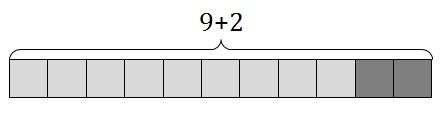# Seeing is Believing

Alignments to Content Standards: 5.OA.A.2

Below is a picture that represents $9 + 2$.1. Draw a picture that represents $4 \times (9 + 2)$.

2. How many times bigger is the value of $4 \times (9 + 2)$ than $9 + 2$? Explain your reasoning.

## IM Commentary

The purpose of this task is to help students see that $4 \times (9 + 2)$ is four times as big as $(9 + 2)$. Though this task may seem very simple, it provides students and teachers with a very useful visual for interpreting an expression without evaluating it because they can see for themselves that $4 \times (9 + 2)$ is four times as big as $(9 + 2)$. Using the two colors also allows students and teachers to see the value of the expression $4 \times (9 + 2)$ as $4\times 9 + 4\times 2$ which would provide a great segue into a discussion of the distributive property.

There are three basic kinds of visual models: set models, length models, and area models. Set models consist of discrete objects; numbers are represented by that number of objects, and multiplication by a whole number is usually represented by specifying a number of groups. Here is how we might represent $4 \times (9 + 2)$ with a set model:Length models represent numbers as lengths and multiplication by a whole number via "scaling" (see 5.OA.5). So, for example, $4 \times (9 + 2)$ would be represented by a line segment or a long, thin rectangle (a.k.a. a tape diagram or a bar diagram) that is four times as long as the segment or rectangle that represents $9+2$. A length model doesn't work as well for the purpose of this task because students are just beginning to interpret multiplication as scaling, and the way this particular argument is represented via length models is best appreciated once students have already come to understand multiplication as scaling very well. However, a student who is proficient with multiplication as scaling might very well draw a length model in response to part (a) and it would be correct (if harder to interpret in this context).

The model suggested by the picture and shown in the solution is an area model. The subtlety with the area model is that the factors are represented by lengths (the lengths of the sides of the rectangle, to be precise), and the product is represented by the area. To really see this, we can label the other side of the rectangle shown in the task statement:If students have colored pencils and graph paper, they could easily draw the picture shown in the solution and could also draw others like $5 \times (9 + 2)$ or $6 \times (9 + 2)$ to really bring the point home.

## Solution

1.2. The value of $4 \times (9+2)$ is four times the value of $9 + 2$. We can see it in the picture since $4 \times (9+2)$ is visually represented as 4 equal rows with $9 + 2$ squares in each row. Without even simplifying each expression, we can see that $4 \times (9+2)$ must be four times the value of $9 + 2$.

We can also verify it by calculating the values of the expressions: $9+2 = 11$ and $4 \times (9+2) = 4 \times 11 = 44$ and then noting that $$44\div 11 = 4$$ But really all we have to do is notice at the step $4 \times (9+2) = 4 \times 11$ that $4 \times (9+2)$ is 4 times $9+2$.